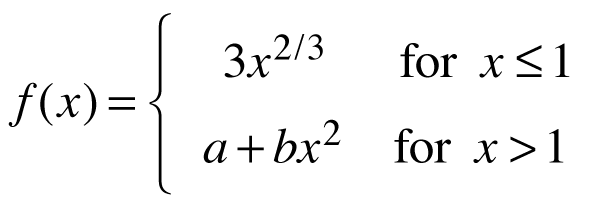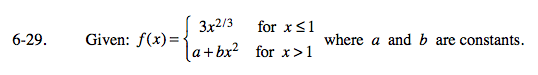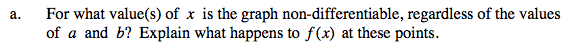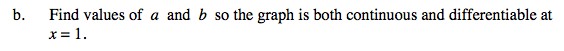### Home > CALC > Chapter 6 > Lesson 6.1.2 > Problem6-29

6-29.
1. Given:where a and b are constants. Homework Help ✎

1. For what value(s) of x is the graph non-differentiable, regardless of the values of a and b? Explain what happens to f(x) at these points.

2. Find values of a and b so the graph is both continuous and differentiable at x = 1.f(x) has a cusp within the domain of one of the pieces. Where is it?Write a system of equations: [piece 1 of f(x)] = [piece 2 of f(x)], evaluated at x = 1. [piece 1 of f '(x)] = [piece 2 of f '(x)], evaluated at x = 1.
Use algebra to solve for a and b.

Use the eTool below to visualize the problem.
Click the link at right for the full version of the eTool: Calc 6-29 HW eTool